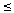Cut The Knot!

An interactive column using Java applets
by Alex Bogomolny

Hearty Munching on Cardioids

September 1998Cardioid (heart-shaped) is a curve with a characteristic shape which is variably described as "vaguely resembling heart", "kidney bean like", or "apple cross-section." The curve was named by Johann Castillon in a treatise that appeared in 1741. At the time, Castillon was 37 and might have still felt light-hearted; perhaps he wrote the treatise in the spring... To me, the curve is distinctly "apple-like" (meeloid might be an appropriate term.) The language of fruits and delicacies become relevant in expectation of a morsel that relates the cardioid at the heart of the Mandelbrot set to the Farey and Fibonacci sequences. In a paper scheduled to appear in The American Mathematical Monthly this Fall, Bob Devaney of Boston University elaborates on the wonderful results described at his web site.

As I digest the paper, I hope to follow suit with a page of my own. Meanwhile, here is an old dish with a new dressing.This applet requires Sun's Java VM 2 which your browser may perceive as a popup. Which it is not. If you want to see the applet work, visit Sun's website at https://www.java.com/en/download/index.jsp, download and install Java VM and enjoy the applet.

Defined kinematicly, cardioid is the locus of points traced by a point (M) on a moving circle that rolls without slipping on the outside of a circle of equal radius (click!).

We start with two circles with centers at O (stationary) and P (moving.) Note three points A, M, and T. Initially they coincide. A remains on the stationary circle. M is the point of the moving circle that traces a cardioid. T is the point of contact of the two circles. The fact that the motion is without slipping is more accurately expressed as the congruence of the corresponding arcs TA and TM of the two circles.

Arc congruence creates a shifting symmetry especially noticeable when the three points are close to each other. The symmetry is obviously with respect to the perpendicular bisector of the segment AM (click!). Because of that symmetry, the bisector is tangent to both circles. The cardioid may thus be described as the locus of all points symmetric to a fixed point (A) on a circle with respect to all possible tangents to that circle.

The midpoints of segments AM lie directly on the tangent lines. We then conclude that the similarity transformation with the center at A and coefficient 1/2 transforms the cardioid into the locus of feet of the perpendiculars dropped from A onto all the tangents of the stationary circle. But this transformation shrinks the whole picture (the circles in particular) so that the shrunk segment AM is half the length of what it used to be, so to speak. Therefore, the image of the cardioid under the transformation is a smaller cardioid, and we get a third definition of the cardioid as the pedal curve of the circle. (The pedal is the locus of the foot of the perpendicular from a fixed point to a variable tangent on a given curve.)

If we move point M towards A even a little, the perpendicular bisector of M will cross the stationary circle. It follows that, for points M0 in the interior of the cardioid (each on its own segment AM), we have TM0TA. M0 is thus inside the circle centered at T with radius TA. We came to the fourth characterization of cardioid as the envelope of the family of circles that pass through a fixed point (A) with centers on a given circle. (Drag the small yellow circle.)

This applet requires Sun's Java VM 2 which your browser may perceive as a popup. Which it is not. If you want to see the applet work, visit Sun's website at https://www.java.com/en/download/index.jsp, download and install Java VM and enjoy the applet.

The motion of the point M is conspicuously not uniform (click!). It lingers near A and accelerates as it moves farther from A. The reason is that M participates in two separate motions. The moving circle rotates around its center P which itself circles around O, the center of the stationary circle. While the point P moves throughAOP =AOT, the point M moves throughTPM. Since the circles move without slippingAOT =TPM. But P moves at the end of the radius OP which is twice as large as PM. Therefore, the angular velocity of M around P is twice that of P around O.

If at all times OQ is equal and parallel to PM, then Q circles around O at the angular speed which is double that of P (click!). This is then yet another method to draw a cardioid. Build a hinged parallelogram OPMQ with OP twice as long as OQ. Make OQ rotate twice as fast as OP. The fourth vertex M of the parallelogram will trace a cardioid.

A small part of the cardioid can be obtained in an even simpler manner. Consider chords QA. Extend each beyond A to the total length of twice the radius of the circle. As Q moves around the circle, the other end M traces a cardioid. To obtain the whole cardioid, double the length of MQ by extending it beyond Q. The second end (click!click!), say N, will trace the same cardioid. (For there were two ways to construct the parallelogram to start with.)

Thus we are reminded of an interesting property of the curve: all chord MN through the point A (the cusp) of the cardioid have the same length which is 4 times the radius of the generating circles. The midpoint Q of such chords traces a circle which, of course, is not the best way to draw it.

References

1. V. Gutenmacher, N. Vasilyev, Lines and Curves: A Practical Geometry Handbook , Birkhauser; 1 edition (July 23, 2004)
2. E.W.Weisstein, The CRC Concise Encyclopedia of Mathematics, CRC Press, 1998